Ex 10.3

Chapter 10 Class 7 Practical Geometry
Serial order wise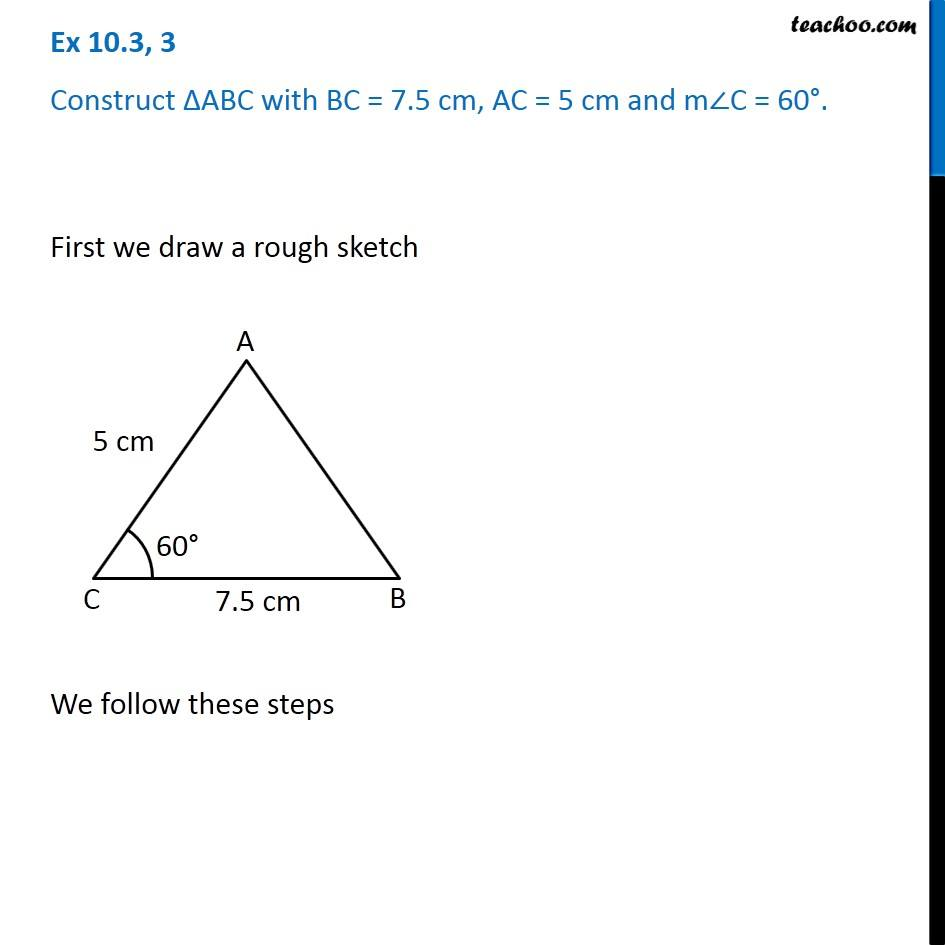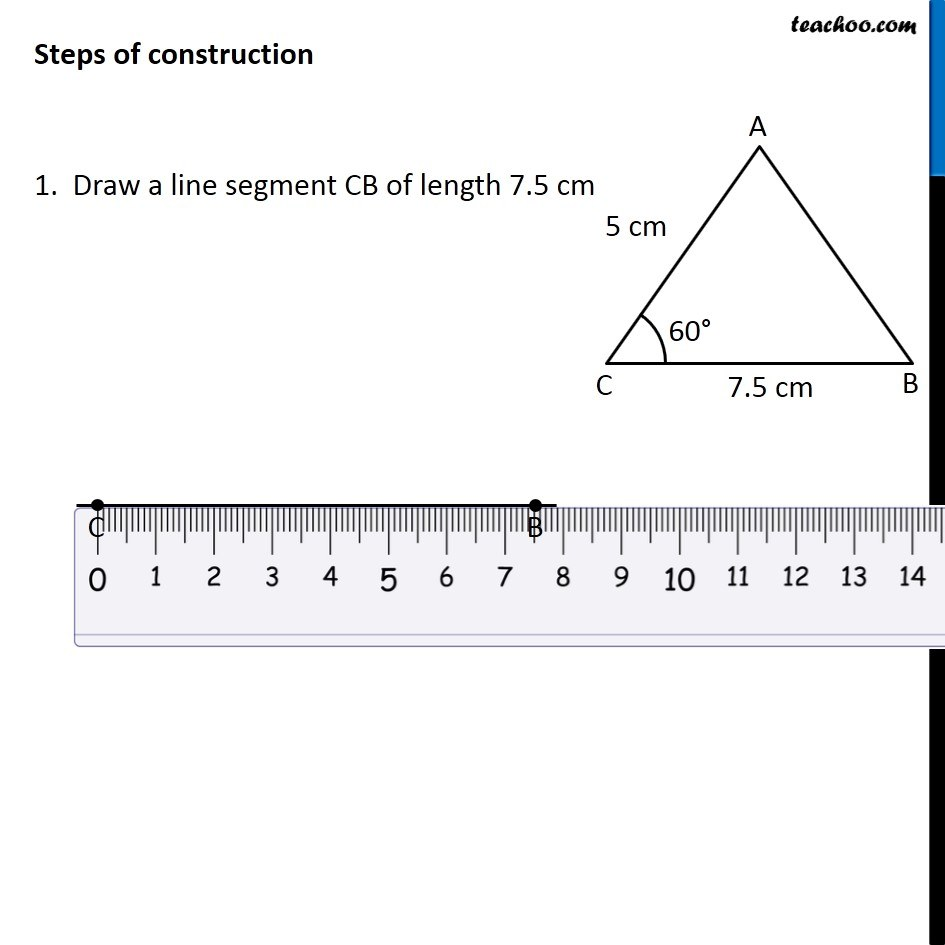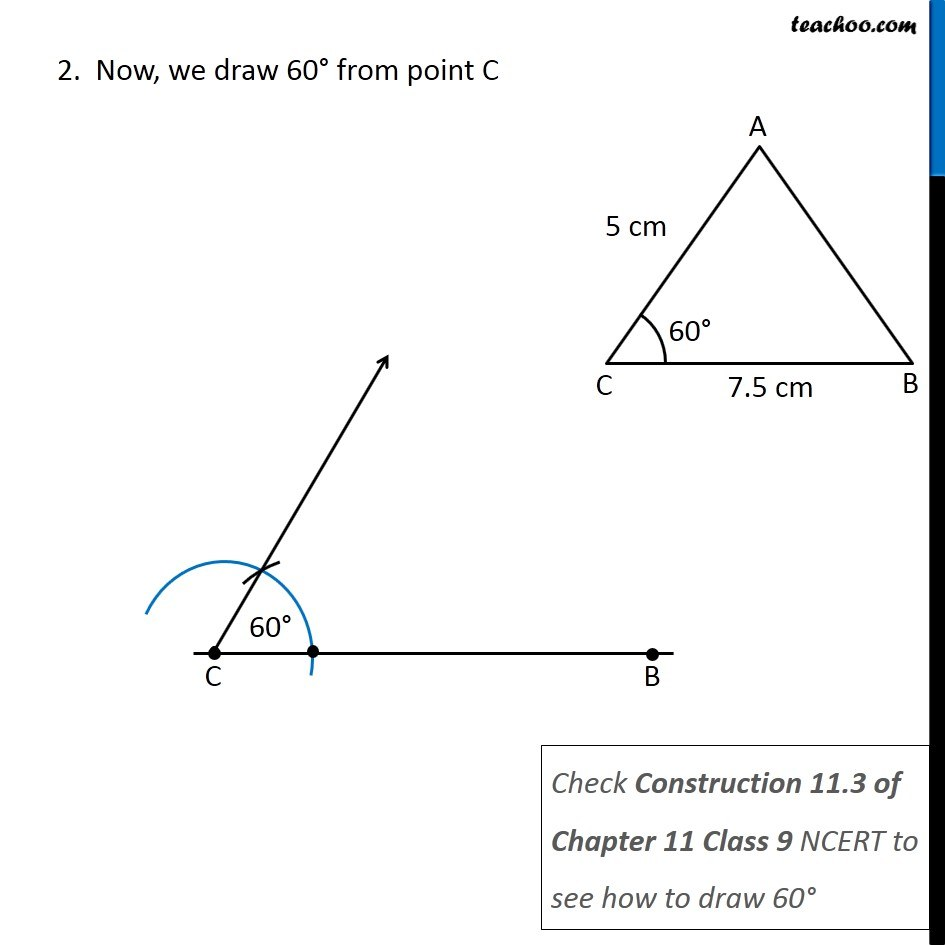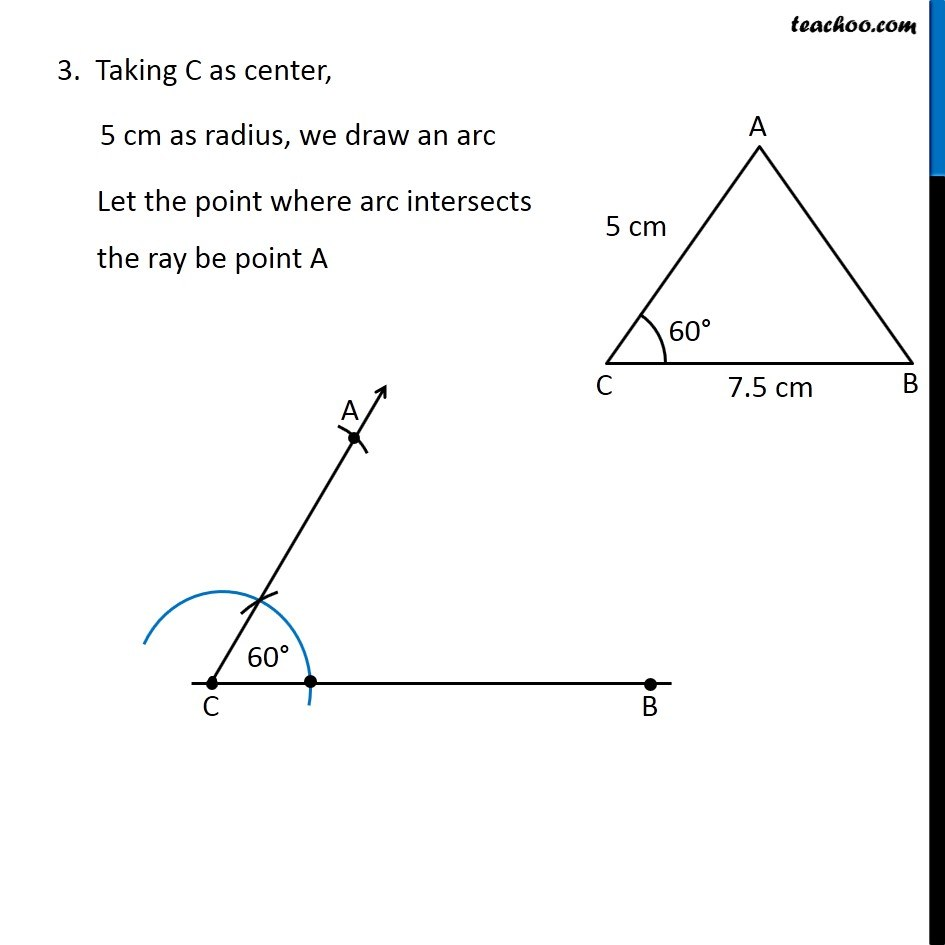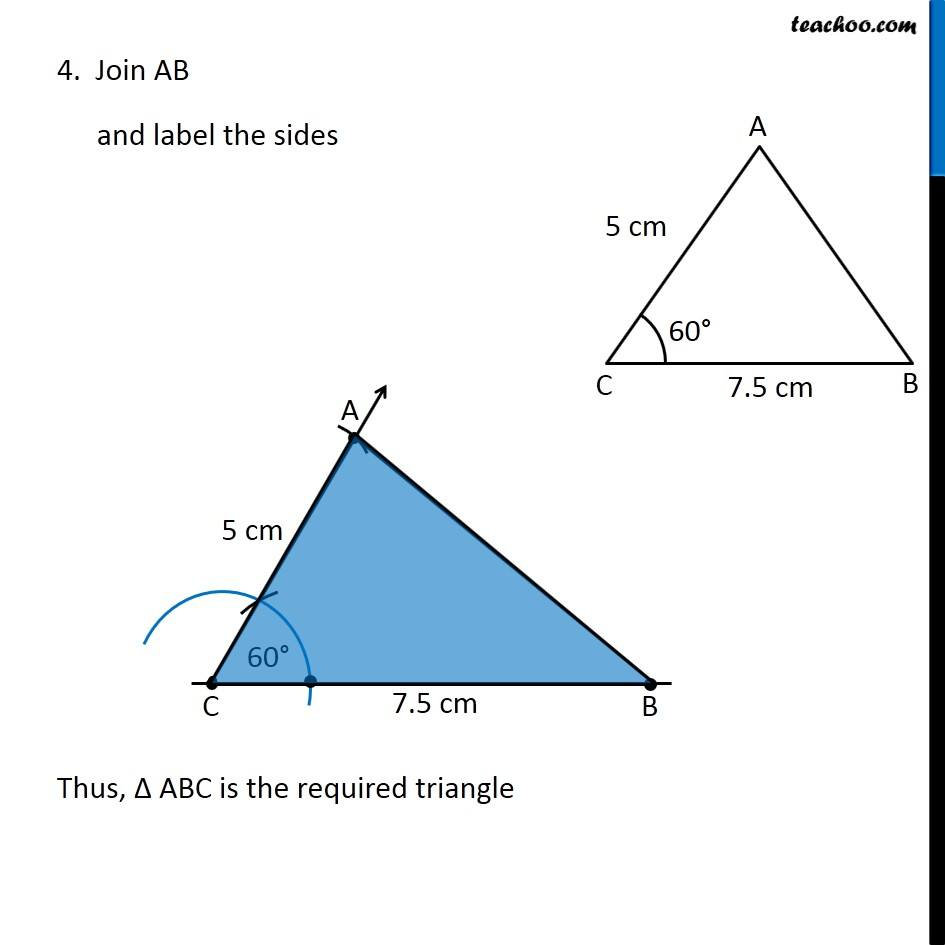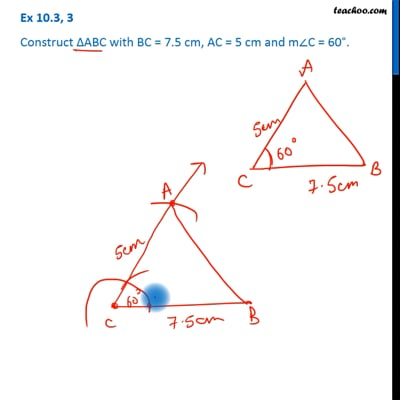This video is only available for Teachoo black users

Get live Maths 1-on-1 Classs - Class 6 to 12

### Transcript

Ex 10.3, 3 Construct ∆ABC with BC = 7.5 cm, AC = 5 cm and m∠C = 60°. First we draw a rough sketch We follow these steps Steps of construction 1. Draw a line segment CB of length 7.5 cm 2. Now, we draw 60° from point C 3. Taking C as center, 5 cm as radius, we draw an arc Let the point where arc intersects the ray be point A 4. Join AB and label the sides Thus, Δ ABC is the required triangle